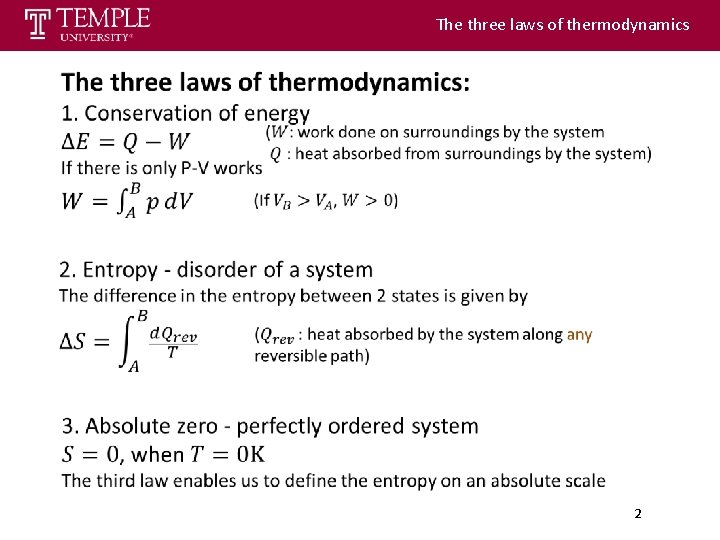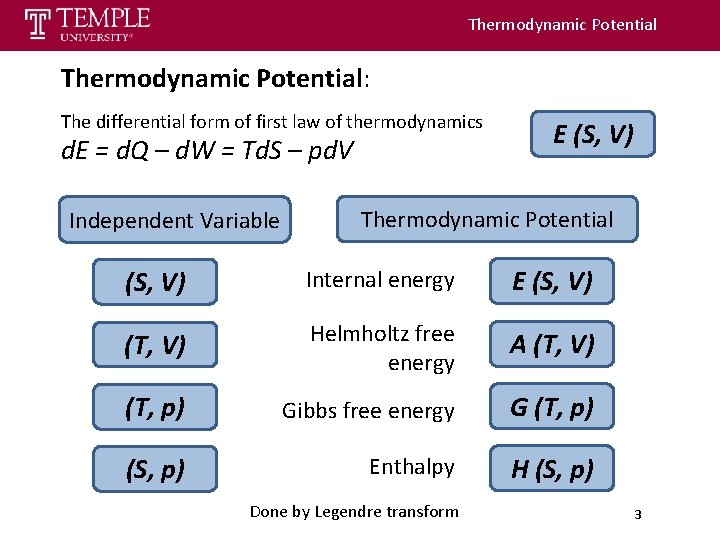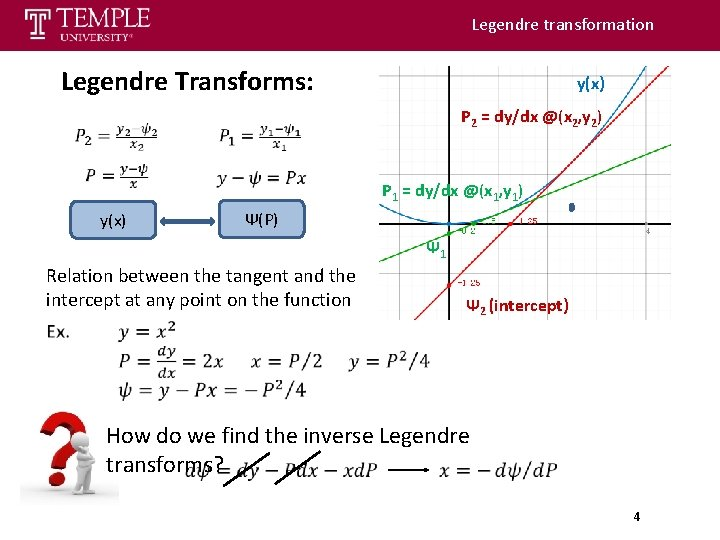# Statistical Thermodynamics Lecture 1 Thermodynamics review Dr Ronald

• Slides: 7Statistical Thermodynamics Lecture 1： Thermodynamics review Dr. Ronald M. Levy [email protected] eduChemical Physics: Statistical mechanics Chemical Physics: explain microscopic properties based on the properties of individual molecules and molecular interactions Statistical Mechanics: Statisitical Mechanics Microscopic Atom Macroscopic Molecule Thermodynamics Themodynamics: Mathematical relation between experimental properties of macroscopic systems MQ. Chapter 1 volumetric thermal Isothermic compressibility expansion coefficient 1The three laws of thermodynamics 2Thermodynamic Potential: The differential form of first law of thermodynamics d. E = d. Q – d. W = Td. S – pd. V Independent Variable E (S, V) Thermodynamic Potential (S, V) Internal energy E (S, V) (T, V) Helmholtz free energy A (T, V) (T, p) Gibbs free energy G (T, p) (S, p) Enthalpy H (S, p) Done by Legendre transform 3Legendre transformation Legendre Transforms: y(x) P 2 = dy/dx @(x 2, y 2) y(x) P 1 = dy/dx @(x 1, y 1) Ψ(P) Ψ 1 Relation between the tangent and the intercept at any point on the function Ψ 2 (intercept) How do we find the inverse Legendre transforms? 4Application of Legendre transform 5Maxwell Relations and Thermodynamic square: V V T S S S P S Symmetric means + T Thermodynamic square F V T P E V P S P T G Asymmetric means - P 1 st Derivative Equation of state S P Gibbs free energy G V T H 2 st Derivative Maxwell relations 6